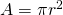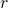# Rearranging Formulae

The area of a circle formula tells us that. Let’s rearrange that to makethe subject. Right now,is the subject.We used our familiar operations: add, subtract, multiply, divide, raise to an exponent, take a root, open brackets, factor etc, to maintain equality while putting the valueon its own on the left hand side.

The circumference of a circle is given by the formula. Rearrange to makethe subject.

A straight line has equation. Rearrange to makethe subject.

A straight line has equation. Rearrange to makethe subject.

Pythagoras theorem tells us that for a right angled triangle,. Nowis the subject of that formula. Rearrange to makethe subject.

The area of a trapezium is given by the formula, whereandare the parallel sides. Rearrange to makethe subject.